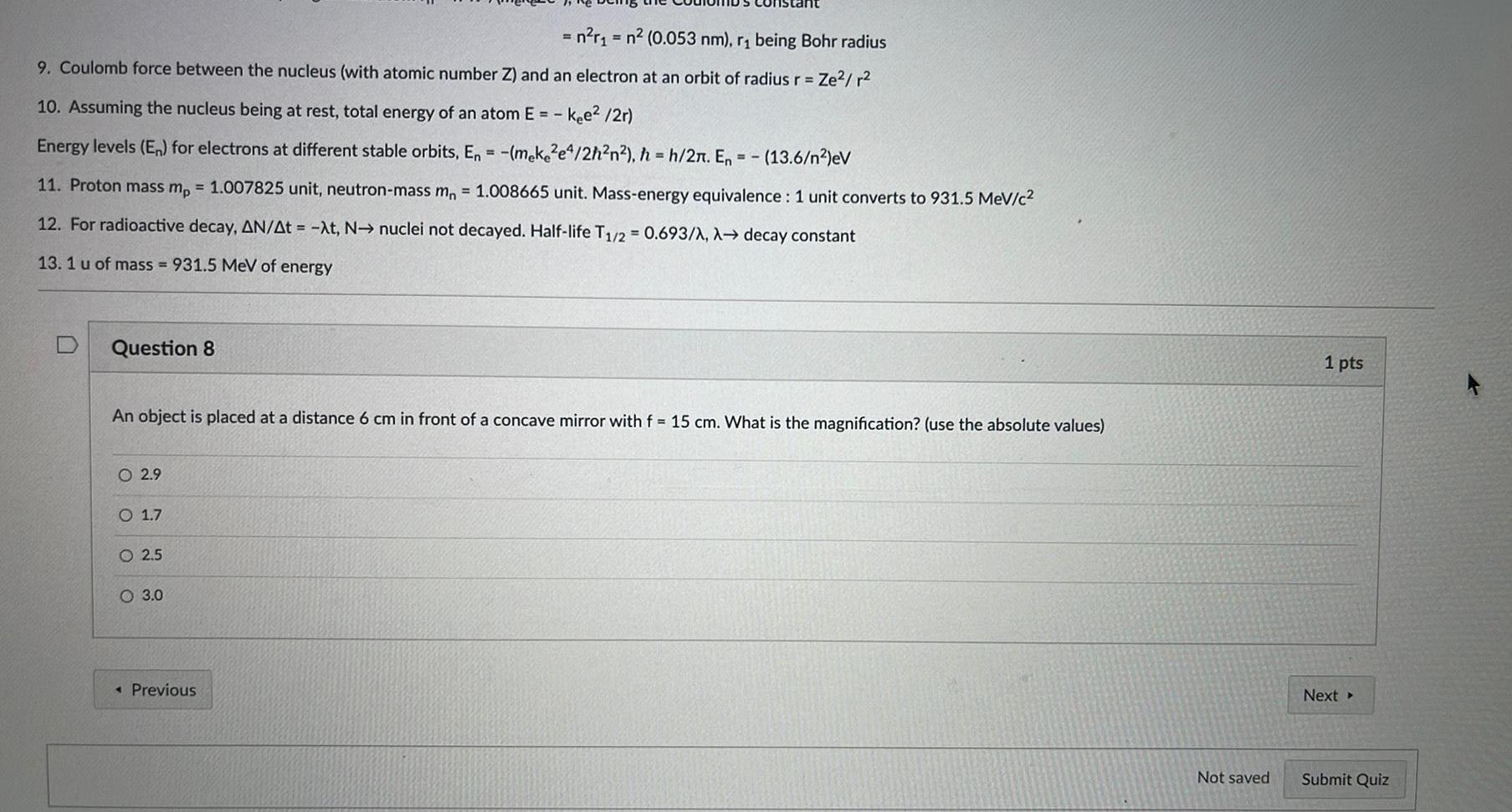Question:

# 9. Coulomb force between the nucleus (with atomic number Z)

Last updated: 8/9/20229. Coulomb force between the nucleus (with atomic number Z) and an electron at an orbit of radius r = Ze²/r² 10. Assuming the nucleus being at rest, total energy of an atom E= - kee²/2r) Energy levels (En) for electrons at different stable orbits, En = -(meke2e4/2h²n²), h=h/2π. En = -(13.6/n²)eV 11. Proton mass mp = 1.007825 unit, neutron-mass mn = 1.008665 unit. Mass-energy equivalence: 1 unit converts to 931.5 MeV/c² 12. For radioactive decay, ΔN/Δt = -λt, N→ nuclei not decayed. Half-life T1/2= 0.693/λ, λ→ decay constant 13. 1 u of mass=931.5 MeV of energy Question 8 An object is placed at a distance 6 cm in front of a concave mirror with f = 15 cm. What is the magnification? (use the absolute values) 2.9 1.7 2.5 3.0